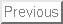An algorithm to estimate the degree of C0-sufficiency of a map germ

by Carles Bivià-Ausina (Univ. Valencia, Spain)

Given an analytic function germ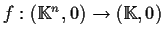, where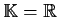or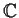, if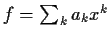is its Taylor expansion, we can consider the problem of determining which is the least integer r such that f is topologically equivalent to the r-jet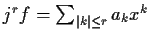, where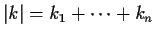, if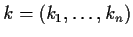. That is, we want to determine the least integer rsuch that there exists a germ of homeomorphism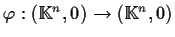with the condition that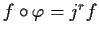. This is called the degree of C0-sufficiency of f and we denote it by s(f). The problem of determining this number is well known in singularity theory.

In this talk, we show an algorithm to give a sharp estimate for s(f), when f is an arbitrary analytic function germ. The difference between the estimate we give and s(f) is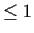. Our method is based on the characterization of s(f) by Chang-Lu, in the complex case, and Bochnak-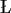ojasiewicz and Kuo, in the real case. This characterization allows us to relate s(f) with the notion of integral closure of an ideal. But the integral closure of an ideal I in a local ring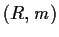is related with the multiplicity e(I), in the Hilbert-Samuel sense (where I is an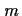-primary ideal). Then we can use the program Singular in order to give an algorithm to determine s(f). Hence we use commutative algebra methods to deal with a problem from real analytic geometry.